Levels »

# Ascension

### The Pyramid, Elevation: 13.6m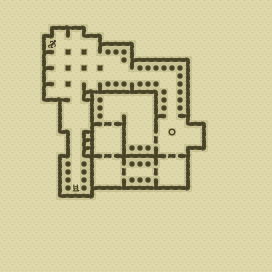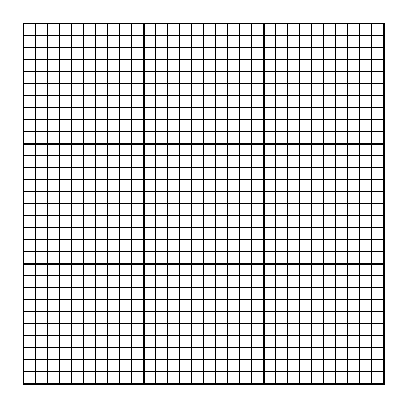### Items

•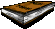Copy of Mein Kampf (5, 4) (on Joachim)
•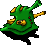Alien Pipes (16, 16)
•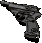Walther P4 Pistol (5, 4) (on Joachim)
•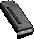8 Walther P4 Magazines (59 rounds) (5, 4) x3 (in Walther P4 Pistol) (on Joachim) - (5, 4) x8 (on Joachim) - (5, 4) x8 (on Joachim) - (5, 4) x8 (on Joachim) - (5, 4) x8 (on Joachim) - (10, 9) x8 - (10, 9) x8 - (23, 15) x8

•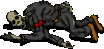(5, 4) Joachim
•Walther P4 Pistol
•Walther P4 Magazine x3
•Walther P4 Magazine x8
•Walther P4 Magazine x8
•Walther P4 Magazine x8
•Walther P4 Magazine x8
•Copy of Mein Kampf

### Monsters

•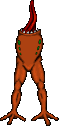23 Headless (Random rate: 10) (8, 3) - (6, 4) - (10, 8) - (6, 10) - (15, 13) - (16, 13) - (17, 13) - (7, 14) - (9, 14) - (16, 14) - (15, 16) - (16, 16) - (16, 16) - (17, 16) - (19, 17) - (21, 17) - (20, 18) - (12, 19) - (13, 19) - (19, 19) - (12, 20) - (11, 21) - (13, 21)
•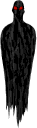4 Phantasms (5, 8) - (15, 14) - (17, 14) - (21, 16)
•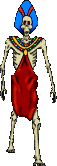15 Zombies (Random rate: 15) (10, 4) - (5, 6) - (9, 6) - (7, 13) - (15, 13) - (9, 14) - (16, 14) - (15, 15) - (15, 15) - (16, 15) - (17, 15) - (23, 15) - (23, 16) - (11, 20) - (12, 21)

### Locations

• 5 Door closers (12, 13) - (12, 17) - (20, 19) - (13, 20) - (17, 20)
• Door opener (17, 16)
• 11 Do-not-disturb sectors(15, 13) - (16, 13) - (17, 13) - (15, 14) - (16, 14) - (17, 14) - (15, 15) - (16, 15) - (17, 15) - (15, 16) - (16, 16)
• Save rune(20, 15)